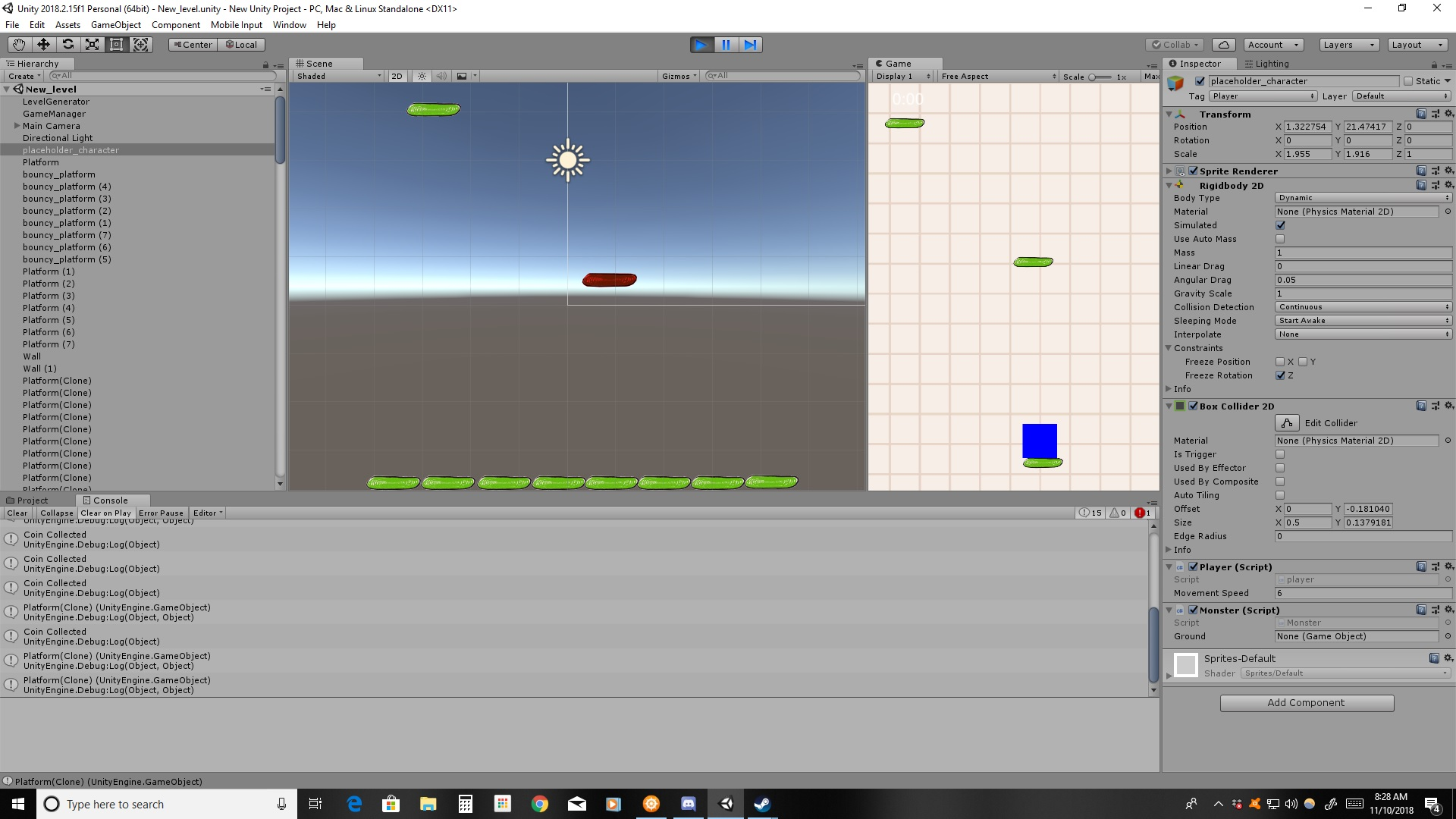Time Stamp Error Help

I'm trying to make a time stamp in the top left corner for my game to emulate an old school anime tv show, but I want it to start at an arbitrary point in time like say "4:00" but no matter what I tell it, it only works at the "00:00" mark.

does anyone know a way to make it at 4 hours in instead of the beginning? Here is my code:

``````using System.Collections;
using System.Collections.Generic;
using UnityEngine;
using UnityEngine.UI;

public class timer : MonoBehaviour {

Text text;
float theTime;
public float speed = 1;

// Use this for initialization
void Start () {
text = GetComponent<Text>();
}

// Update is called once per frame
void Update () {
theTime += Time.deltaTime * speed;
string hours = Mathf.Floor((theTime % 216000) / 3600).ToString("0");
string minutes = Mathf.Floor((theTime % 3600)/60).ToString("00");
string seconds = (theTime % 60).ToString("00");
text.text = hours + ":" + minutes;
}
}
``````

Screen shot of my game view.• At first glance, it doesn't show me anywhere where you would start off at a random value like 4:00. Your theTime variable is set to 0 by default that's why it always starts off that way. You can change it in Start randomly with something like this:

``````theTime = Random.Range(0, 10);
``````

Then whenever you play the game it'll randomly give it a value between 0 and 10, but note this will also change the values for the hours/minutes/seconds as well. If that's all you wanted then that should be an easy fix.

• Not sure if I understand the problem. But asjgonzalez says, just add a start value to theTime and it should work. If it constantly  shows zeroes you have probably set speed = 0 somewhere.

Anyway. I'd suggest using System.TimeSpan for calculations like this, it makes everything simpler and more readable.

``````public class timer: MonoBehaviour
{

private Text text;
private TimeSpan theTime;
public float speed = 1;

void Start()
{
text = GetComponent<Text>();
theTime = new TimeSpan(hoursToAdd, 0, 0);
}

void Update()
{